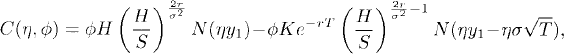# PATH-DEPENDENT OPTIONS

Path-dependent options are also referred to as “exotic options”, whose payoffs depend on the path of the underlying asset. In this chapter, we present pricing formulas for some path-dependent options within the Black-Scholes framework.

# 43.1 Basic Concepts and Facts

Theorem 43.1 (European Barrier Option Price). Let {(X(0)t, Xt) : 0 ≤ tT} be the Black-Scholes market given in Definition 42.2. Let

(43.1a)(43.1b)(43.1c)and

(43.1d)where S = X0 is the initial price of the risky asset, K is the strike, T is the expiration time, H is the barrier, N(·) is as defined in Equation (42.1c), andThen

(a) If S > H and the payoff of a down-and-in call isthen the price at time 0 of the down-and-in call is

(43.2)(b) If S < H and the payoff of an up-and-in call ...

Get Measure, Probability, and Mathematical Finance: A Problem-Oriented Approach now with the O’Reilly learning platform.

O’Reilly members experience books, live events, courses curated by job role, and more from O’Reilly and nearly 200 top publishers.